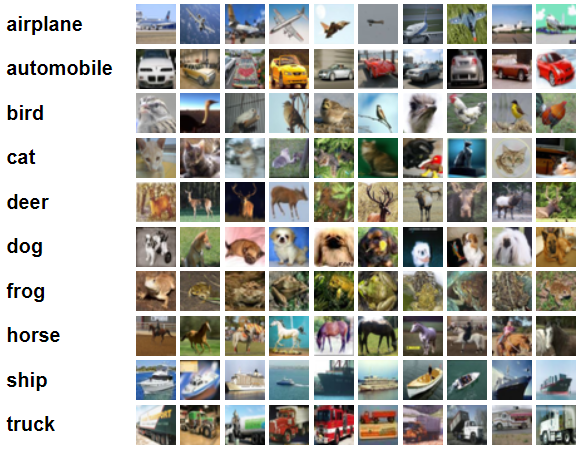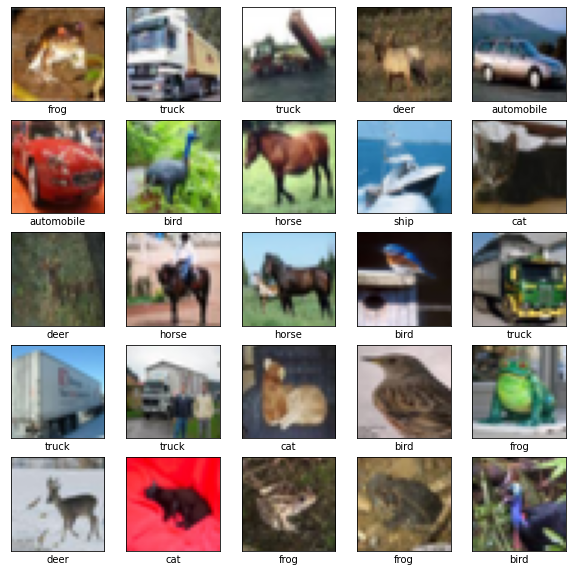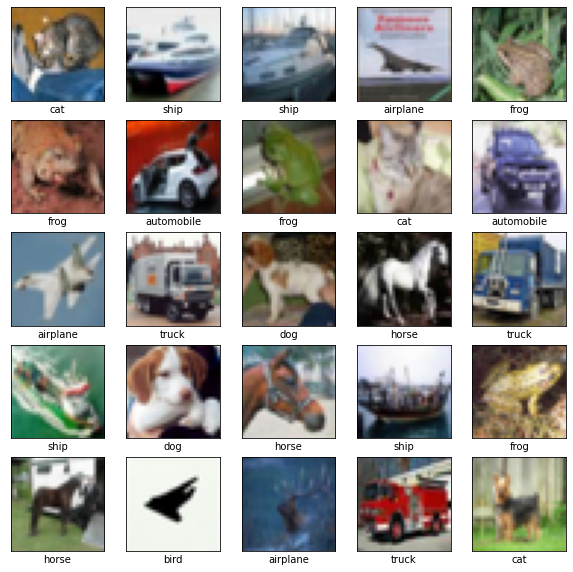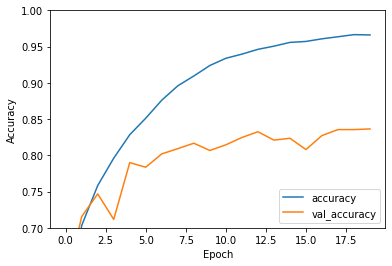### Image Classification with CIFAR10 Dataset using Tensorflow Keras | DL4CV -1

This is a first post of the Deep Learning for Computer Vision (DL4CV) tutorial series.  For different types of the computer vision problems, deep learning techniques have achieved high accuracy. Image classification is a well defined problem in image processing and computer vision. We are going to perform image classification using a well known deep learning technique - CNN (Convolutional Neural Network). The CNNs are very useful for to perform image processing and computer vision related tasks efficiently. We will use CIFAR 10 dataset for training and testing the CNN model. Let's take a deep dive into the steps to understand how the image classification works. In this project, we will cover the following topics.

### Prerequisites

Below are the prerequisites for this project.

• Language: Python 3 or Higher
• Frameworks: TensorFlow
• Libraries: NumPy, Matplotlib and scikit-learn
We will be using Python as our main programming language. We used python 3.6 for this project.
We are going to use TensorFlow- an open source framework for machine learning and Artificial Intelligence.
We also use matplotlib for image visualization purpose. It is is a comprehensive library for creating  interactive, static,and animated visualizations in Python.
We use scikit-learn to perform analysis of the results ( precision recall f1-score support, confusion matrix etc).
NumPy is obvious to use to perform any mathematical task.

## Import Required Libraries

Now we import all the required libraries and framework.

`import tensorflow as tffrom tensorflow.keras import datasets, layers, modelsimport matplotlib.pyplot as pltfrom sklearn.metrics import confusion_matrix , classification_reportimport numpy as np`
We import tensorflow as tf. Also the image datasets, CNN layers and models are imported from tensorflow.keras. Here Keras is a deep learning API written is Python. We different scikit-learn metrics from sklearn.metrics. The libraries Matplotlib and NumPy also imported as well.

After importing the required libraries and frameworks, the next task is to load the CIFAR 10 dataset.
There are total 10 classes in the dataset.
`["airplane","automobile","bird","cat","deer","dog","frog","horse","ship","truck"]`CIFAR-10 Images with 10 ClassesImage Source: https://www.cs.toronto.edu/~kriz/cifar.html

The dataset is loaded as train and test datasets. Below code snippet is used to load the dataset.
`(X_train, y_train), (X_test, y_test) = datasets.cifar10.load_data()X_train.shape`

#### Output

`Downloading data from https://www.cs.toronto.edu/~kriz/cifar-10-python.tar.gz 170500096/170498071 [==============================] - 3s 0us/step 170508288/170498071 [==============================] - 3s 0us/step(50000, 32, 32, 3)`
You can notice that the total images in the train dataset is 50,000. The size of each image is (32,32,3). The image height and width is 32 each having 3 channels- RGB.

You can print the size of the test dataset the same as we printed train dataset. Use X_test.shape to find the shape/ size of test dataset.
`X_test.shape`
`(10000, 32, 32, 3)`
There are 10,000 images in test dataset each having the size of (32,32,3) as the same train dataset.
The same way we can find the shape of training and test labels.
`y_train.shape`
`(50000, 1)`
`y_test.shape`
`(10000, 1)`

How to Load, Pre-process and Visualize CIFAR-10 and CIFAR -100 datasets in Python

## Visualize Random Images from CIFAR10 Dataset

Now we have loaded the train and test datasets, let's visualize some images to understand how the images from CIFAR 10 datasets look like.
`class_names = ['airplane', 'automobile', 'bird', 'cat', 'deer', 'dog', 'frog', 'horse', 'ship', 'truck']plt.figure(figsize=(10,10))for i in range(25):    plt.subplot(5,5,i+1)    plt.xticks([])    plt.yticks([])    plt.grid(False)    plt.imshow(train_images[i])    # The CIFAR labels happen to be arrays,     # which is why you need the extra index    plt.xlabel(class_names[train_labels[i]])plt.show()`

#### OutputBefore moving to next step to define the Convolutional Neural Network, let's normalize train and test dataset.
`X_train = X_train / 255.0X_test = X_test / 255.0`
Now the train and test datasets are normalized, we should move to define the Convolutional Neural Network.

## Define a Convolutional Neural Network (CNN)

We have our train dataset ready, we should define a Convolutional Neural Network (CNN). We use a sequential model to define our CNN. We use a sequence of different layers such Convolution 2D layer, Batch Normalization layer, Max pooling 2D layer, flatten, dropout and dense layers. We use 'relu' as activation function.
`# number of classesy_train = y_train.reshape(-1,)K = len(set(y_train))# calculate total number of classes# for output layerprint("number of classes:", K)# Build the model using the functional API# input layermodel = models.Sequential()model.add(layers.Conv2D(32, (3, 3), activation='relu', padding='same', input_shape=(32, 32, 3)))model.add(layers.BatchNormalization())model.add(layers.Conv2D(32, (3, 3), activation='relu', padding='same'))model.add(layers.BatchNormalization())model.add(layers.MaxPooling2D((2, 2)))model.add(layers.Conv2D(64, (3, 3), activation='relu', padding='same'))model.add(layers.BatchNormalization())model.add(layers.Conv2D(64, (3, 3), activation='relu', padding='same'))model.add(layers.BatchNormalization())model.add(layers.MaxPooling2D((2, 2)))model.add(layers.Conv2D(128, (3, 3), activation='relu', padding='same'))model.add(layers.BatchNormalization())model.add(layers.Conv2D(128, (3, 3), activation='relu', padding='same'))model.add(layers.BatchNormalization())model.add(layers.MaxPooling2D((2, 2)))model.add(layers.Flatten())model.add(layers.Dropout(0.2))# Hidden layermodel.add(layers.Dense(1024, activation='relu'))model.add(layers.Dropout(0.2))# last hidden layer i.e.. output layermodel.add(layers.Dense(K, activation='softmax'))# model descriptionmodel.summary()`

#### Output

```number of classes: 10
Model: "sequential"
_________________________________________________________________
Layer (type)                Output Shape              Param #
=================================================================
conv2d (Conv2D)             (None, 32, 32, 32)        896

batch_normalization (BatchN  (None, 32, 32, 32)       128
ormalization)

conv2d_1 (Conv2D)           (None, 32, 32, 32)        9248

batch_normalization_1 (Batc  (None, 32, 32, 32)       128
hNormalization)

max_pooling2d (MaxPooling2D  (None, 16, 16, 32)       0
)

conv2d_2 (Conv2D)           (None, 16, 16, 64)        18496

batch_normalization_2 (Batc  (None, 16, 16, 64)       256
hNormalization)

conv2d_3 (Conv2D)           (None, 16, 16, 64)        36928

batch_normalization_3 (Batc  (None, 16, 16, 64)       256
hNormalization)

max_pooling2d_1 (MaxPooling  (None, 8, 8, 64)         0
2D)

conv2d_4 (Conv2D)           (None, 8, 8, 128)         73856

batch_normalization_4 (Batc  (None, 8, 8, 128)        512
hNormalization)

conv2d_5 (Conv2D)           (None, 8, 8, 128)         147584

batch_normalization_5 (Batc  (None, 8, 8, 128)        512
hNormalization)

max_pooling2d_2 (MaxPooling  (None, 4, 4, 128)        0
2D)

flatten (Flatten)           (None, 2048)              0

dropout (Dropout)           (None, 2048)              0

dense (Dense)               (None, 1024)              2098176

dropout_1 (Dropout)         (None, 1024)              0

dense_1 (Dense)             (None, 10)                10250

=================================================================
Total params: 2,397,226
Trainable params: 2,396,330
Non-trainable params: 896
_________________________________________________________________```
Notice total, trainable and non-trainable parameters in the network.

Now we defined successfully our CNN model. Let's move to define the loss function and optimizer.

## Define a Loss Function And an Optimizer

We will be using 'adam' as optimizer and 'sparse_categorical_crossentropy' as loss function.

loss = 'sparse_categorical_crossentropy'

#### Compile the Model

We set the optimizer and the loss function. Now we compile the model.
`model.compile(optimizer='adam',loss = 'sparse_categorical_crossentropy',metrics=['accuracy'])`

Now we have set the loss function and optimizer, we can move to train our network.

## Train the Network on Training Data

We have defined our model and set the loss function and optimizer. Now we train our model network on the training data. We have already loaded and normalized dataset. The below code snippet train our model:
`history = model.fit(X_train, y_train, epochs=20, validation_data=(X_test, y_test))`

#### Output

```Epoch 1/20
1563/1563 [==============================] - 42s 21ms/step - loss: 1.3265 - accuracy: 0.5468 - val_loss: 1.0588 - val_accuracy: 0.6208
Epoch 2/20
1563/1563 [==============================] - 32s 21ms/step - loss: 0.8544 - accuracy: 0.7032 - val_loss: 0.8160 - val_accuracy: 0.7154
Epoch 3/20
1563/1563 [==============================] - 30s 19ms/step - loss: 0.6999 - accuracy: 0.7583 - val_loss: 0.7447 - val_accuracy: 0.7468
Epoch 4/20
1563/1563 [==============================] - 31s 20ms/step - loss: 0.5927 - accuracy: 0.7961 - val_loss: 0.8862 - val_accuracy: 0.7116
Epoch 5/20
1563/1563 [==============================] - 32s 20ms/step - loss: 0.5013 - accuracy: 0.8284 - val_loss: 0.6328 - val_accuracy: 0.7899
Epoch 6/20
1563/1563 [==============================] - 32s 21ms/step - loss: 0.4335 - accuracy: 0.8512 - val_loss: 0.7026 - val_accuracy: 0.7836
Epoch 7/20
1563/1563 [==============================] - 32s 21ms/step - loss: 0.3595 - accuracy: 0.8761 - val_loss: 0.6304 - val_accuracy: 0.8020
Epoch 8/20
1563/1563 [==============================] - 32s 21ms/step - loss: 0.3076 - accuracy: 0.8959 - val_loss: 0.6027 - val_accuracy: 0.8092
Epoch 9/20
1563/1563 [==============================] - 33s 21ms/step - loss: 0.2648 - accuracy: 0.9094 - val_loss: 0.6441 - val_accuracy: 0.8167
Epoch 10/20
1563/1563 [==============================] - 43s 28ms/step - loss: 0.2249 - accuracy: 0.9239 - val_loss: 0.6653 - val_accuracy: 0.8067
Epoch 11/20
1563/1563 [==============================] - 33s 21ms/step - loss: 0.1943 - accuracy: 0.9339 - val_loss: 0.6418 - val_accuracy: 0.8145
Epoch 12/20
1563/1563 [==============================] - 44s 28ms/step - loss: 0.1755 - accuracy: 0.9396 - val_loss: 0.6752 - val_accuracy: 0.8246
Epoch 13/20
1563/1563 [==============================] - 35s 23ms/step - loss: 0.1570 - accuracy: 0.9462 - val_loss: 0.6706 - val_accuracy: 0.8325
Epoch 14/20
1563/1563 [==============================] - 42s 27ms/step - loss: 0.1499 - accuracy: 0.9506 - val_loss: 0.7519 - val_accuracy: 0.8210
Epoch 15/20
1563/1563 [==============================] - 33s 21ms/step - loss: 0.1345 - accuracy: 0.9558 - val_loss: 0.6644 - val_accuracy: 0.8235
Epoch 16/20
1563/1563 [==============================] - 32s 21ms/step - loss: 0.1291 - accuracy: 0.9571 - val_loss: 0.8130 - val_accuracy: 0.8080
Epoch 17/20
1563/1563 [==============================] - 33s 21ms/step - loss: 0.1154 - accuracy: 0.9607 - val_loss: 0.7475 - val_accuracy: 0.8273
Epoch 18/20
1563/1563 [==============================] - 33s 21ms/step - loss: 0.1097 - accuracy: 0.9636 - val_loss: 0.7086 - val_accuracy: 0.8355
Epoch 19/20
1563/1563 [==============================] - 31s 20ms/step - loss: 0.1001 - accuracy: 0.9665 - val_loss: 0.7216 - val_accuracy: 0.8355
Epoch 20/20
1563/1563 [==============================] - 30s 19ms/step - loss: 0.1011 - accuracy: 0.9661 - val_loss: 0.7446 - val_accuracy: 0.8364```

We train our network for 20 epochs. You can train for hundreds of epochs. Notice the accuracy is increasing slowly for higher epochs.

We get the training accuracy of 96.61% and the validation accuracy of 83.64%.

You may increase these accuracies by training the model for more epochs. You may use use data augmentation also. This also helps achieving better validation accuracy using the technique of data augmentation. Data augmentation is a technique for data pre-processing. Using this technique we generate different types of images by transforming the images available in the dataset.

Here we have completed the training part of the model. Now move to test or evaluate our network/ model.

## Test/ Evaluate the Network on Test Data

Now evaluate the model on the test data. Find the predicted scores and classes of test dataset using model.predict(X_test).

`y_pred = model.predict(X_test)y_pred_classes = [np.argmax(element) for element in y_pred]`
Let's print the test scores for each class and for all images in the test dataset.
`y_pred`

#### Output:

```
[[3.9521787e-08 2.3015059e-09 4.6826610e-08 ... 1.2385266e-07
9.0896992e-07 1.3537279e-07]
[1.9760818e-19 1.0568182e-09 1.0820759e-28 ... 4.4578180e-30
1.0000000e+00 1.6756009e-20]
[2.0620810e-05 4.2299676e-04 4.2718745e-11 ... 3.5415070e-08
9.9945992e-01 1.6858961e-06]
...
[8.3391088e-15 6.5921789e-14 1.1535729e-08 ... 4.8390071e-08
1.4339907e-13 4.2596921e-13]
[9.9146479e-01 9.9901354e-04 1.1814365e-03 ... 4.7673228e-05
5.9958766e-03 1.1494376e-05]
[1.4169767e-16 7.0683491e-16 2.0917616e-15 ... 1.0000000e+00
3.8461754e-17 8.3701241e-14]]
```

Lets understand what does the above output means. As we know the test dataset has 10,000 images and we have 10 classes in CIFAR-10 dataset. Each row in the above output shows the predicted scores for all ten classes. We find the maximum of these ten scores to find the predicted class label.

Now print the predicted class labels.
`y_pred_classes`

#### Output:

[3, 8, 8,..., 5, 0, 7]
Notice that above values are the indices of max values in y_pred scores in each rows.
The predicted classes ranges from 0 to 9 for class labels from "airplane" to "truck". Here 3 is the class of "cat". Below is labels of different classes:
`["airplane","automobile","bird","cat","deer","dog","frog","horse","ship","truck"]`

### Predict Some Result

We will predict some images from test dataset. We display the images and corresponding predicted class labels.
`labels = ['airplane', 'automobile', 'bird', 'cat', 'deer', 'dog', 'frog', 'horse', 'ship', 'truck']y_pred = model.predict(X_test)print(y_pred)y_classes = [np.argmax(element) for element in y_pred]//print(y_classes)plt.figure(figsize=(10,10))for i in range(25):     plt.subplot(5,5,i+1)     plt.xticks([])     plt.yticks([])     plt.grid(False)     plt.imshow(X_test[i])     # The CIFAR labels happen to be arrays,      # which is why you need the extra index     # print(y_train[i])     # plt.xlabel("True class:{} "'\n Predicted class: {}' ,labels[y_train[i]], )     plt.xlabel(labels[y_classes[i]])plt.show()`

#### Ouput25 Images with predicted class labels
Notice that most of image labels are predicted correctly.

### Plot Accuracy

Use below code snippet to plot accuracies
`plt.plot(history.history['accuracy'], label='accuracy')plt.plot(history.history['val_accuracy'], label = 'val_accuracy')plt.xlabel('Epoch')plt.ylabel('Accuracy')plt.ylim([0.7, 1])plt.legend(loc='lower right')test_loss, test_acc = model.evaluate(X_test,  y_test, verbose=2)`

#### Output

`313/313 - 2s - loss: 0.7446 - accuracy: 0.8364 - 2s/epoch - 7ms/step`training and validation accuracies

Analyze the two accuracies- training and validation.

### Classification Report

We will find the classification report. You can find the precision, recall, f1-score and support.
`print("Classification Report: \n", classification_report(y_test, y_pred_classes))`

#### Output:

```Classification Report:
precision    recall  f1-score   support

0       0.83      0.88      0.85      1000
1       0.93      0.93      0.93      1000
2       0.77      0.76      0.77      1000
3       0.65      0.73      0.69      1000
4       0.84      0.80      0.82      1000
5       0.81      0.75      0.78      1000
6       0.90      0.85      0.87      1000
7       0.89      0.85      0.87      1000
8       0.86      0.93      0.89      1000
9       0.92      0.89      0.90      1000

accuracy                           0.84     10000
macro avg       0.84      0.84      0.84     10000
weighted avg       0.84      0.84      0.84     10000```

### Confusion Matrix

Find the confusion matrix as below:
`print("confusion matrix:\n", confusion_matrix(y_test, y_pred_classes))`

#### Output:

```confusion matrix:
[[879   4  31  12   4   4   3   6  44  13]
[  9 934   2   2   1   1   2   1  18  30]
[ 48   7 763  53  43  33  26  16   8   3]
[ 19   6  49 731  33  80  33  23  20   6]
[ 18   3  55  54 796  18  17  33   6   0]
[  9   1  27 153  25 747   7  23   4   4]
[ 11   3  30  50  19  16 846   3  17   5]
[ 10   1  25  49  22  26   1 855   6   5]
[ 37  10   7   5   2   1   2   0 926  10]
[ 21  40   5  16   1   1   0   0  29 887]]```
We completed all the point listed in the table of content. It is the first post in the series Deep Learning for Computer Vision (DL4CV). We published "Visualizing Filters and Feature Maps in CNNs using TensorFlow Keras" as our next post.

### Computer VisionHow TO

1.